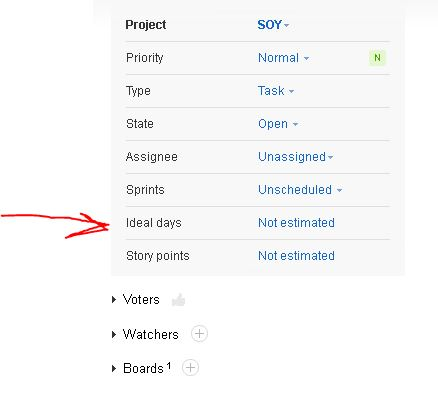How can I get the values from an issues custom fields?  I can do everything but get the info from the custom fields.Liubov Melnikova
Official comment

If you run the GET issue REST request, then all the fields' states will be there, except empty ones.I have been pretty happy with this so far but this is pretty odd.  So for me to get a field I have to loop this whole array and look for the name of the field then get the info out of that field because of the number of items in the array changes depending on if anything is in the custom fields.

```SimpleXMLElement Object
(
[@attributes] => Array
(
[id] => xxxx
[entityId] => 89-24870
)

[field] => Array
(
 => SimpleXMLElement Object
(
[@attributes] => Array
(
[name] => projectShortName
)

[value] => xxx
)

 => SimpleXMLElement Object
(
[@attributes] => Array
(
[name] => numberInProject
)

[value] => 260
)

 => SimpleXMLElement Object
(
[@attributes] => Array
(
[name] => summary
)

[value] => xxx
)

 => SimpleXMLElement Object
(
[@attributes] => Array
(
[name] => description
)

[value] => xxx

)

 => SimpleXMLElement Object
(
[@attributes] => Array
(
[name] => created
)

[value] => 1518115614440
)

 => SimpleXMLElement Object
(
[@attributes] => Array
(
[name] => updated
)

[value] => 1518641067795
)

 => SimpleXMLElement Object
(
[@attributes] => Array
(
[name] => updaterName
)

[value] => xxx
)

 => SimpleXMLElement Object
(
[@attributes] => Array
(
[name] => updaterFullName
)

[value] => xxx
)

 => SimpleXMLElement Object
(
[@attributes] => Array
(
[name] => reporterName
)

[value] => xxx
)

 => SimpleXMLElement Object
(
[@attributes] => Array
(
[name] => reporterFullName
)

[value] => xxx
)

 => SimpleXMLElement Object
(
[@attributes] => Array
(
)

[value] => 10
)

 => SimpleXMLElement Object
(
[@attributes] => Array
(
)

[value] => 0
)

 => SimpleXMLElement Object
(
[@attributes] => Array
(
[name] => Assignee
)

[value] => xxx
)

 => SimpleXMLElement Object
(
[@attributes] => Array
(
[name] => Date of Service
)

[value] => 1518674400000
[valueId] => 1518674400000
)

 => SimpleXMLElement Object
(
[@attributes] => Array
(
[name] => Time Of Service
)

[value] => 20:00
[valueId] => 20:00
)

 => SimpleXMLElement Object
(
[@attributes] => Array
(
[name] => ONSITE
)

[value] => 12:00
[valueId] => 12:00
)
```